Time Value of Money - Six Functions of a Dollar
Using Assessors Handbook Section 505 (Capitalization Formulas and Tables)
Appraisal Training: Self-Paced Online Learning Session

# Lesson 3: Present Worth of \$1(Assessors’ Handbook 505, Column 4)

This lesson discusses the Present Worth of \$1 (PW\$1); one of six compound interest functions presented in Assessors’ Handbook Section 505 (AH 505), Capitalization Formulas and Tables. The Lesson:

• Explains the function’s meaning and purpose,
• Provides the formula for the calculation of PW\$1 factor,
• Shows how to calculate the present value of multiple payments, and
• Contains practical examples of how to apply the PW\$1 factor.

## PW\$1: Meaning and Purpose

The PW\$1 factor is the amount that must be deposited today to grow to \$1 in the future, given periodic interest rate i and n periods.

The PW\$1 factor is used to discount a single future amount to its present amount. The PW\$1 factors are in column 4 of AH 505.

The PW\$1 factor can be thought of as the opposite of the FW\$1 factor; mathematically, the PW\$1 and FW\$1 factors are reciprocals: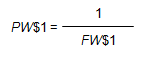Whereas the FW\$1, discussed in Lesson 1, provides the future value of a single present amount, the PW\$1 provides the present value of a single future amount.

The value of the PW\$1 factor will always be less than \$1, explicitly demonstrating that a dollar to be received in the future is worth less than a dollar today.

## Formula for Calculating PW\$1 Factors

The formula for the calculation of the PW\$1 factors is: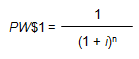Where:

• PW\$1 = Present Worth of \$1 Factor
• i = Periodic Interest Rate, often expressed as an annual percentage rate
• n = Number of Periods, often expressed in years

In order to calculate the PW\$1 factor for 4 years at an annual interest rate of 6%, use the formula below:Viewed on a timeline: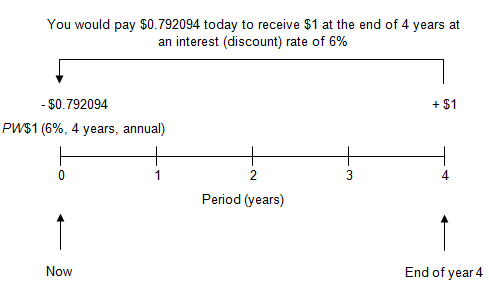A depositor would be willing to give up \$0.792094 today (shown as negative on the timeline) in order to receive \$1 at the end of 4 years (shown as positive).

To locate the factor, go to page 33 of AH 505, go down 4 years, and then across to column 4. The correct PW\$1 factor is 0.792094.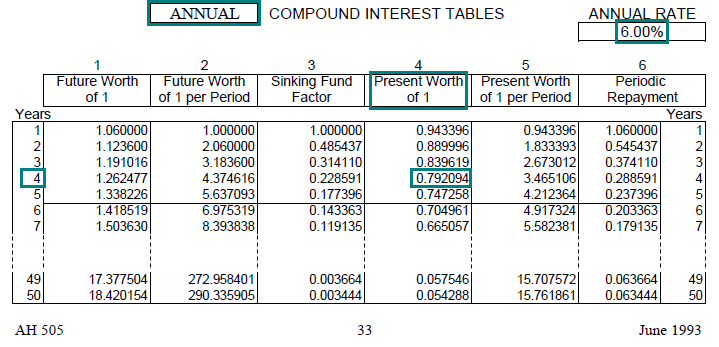Link to AH 505, page 33

## Practical Applications of PW\$1

Example 1:
How much must be deposited today in order to have \$15,000 at the end of 10 years, assuming an annual interest rate of 7% with annual compounding?

Solution:

• PV = FV × PW\$1 (7%, 10 yrs, annual)
• PV = \$15,000 × 0.508349
• PV = \$7,625
• Find the annual PW\$1 factor (annual compounding) for 7% at a term of 10 years. In AH 505, page 37, go down 10 years and across to column 4 to find the correct factor of 0.508349.
• The present value of \$7,625 is equal to the future value of \$15,000 multiplied by the factor.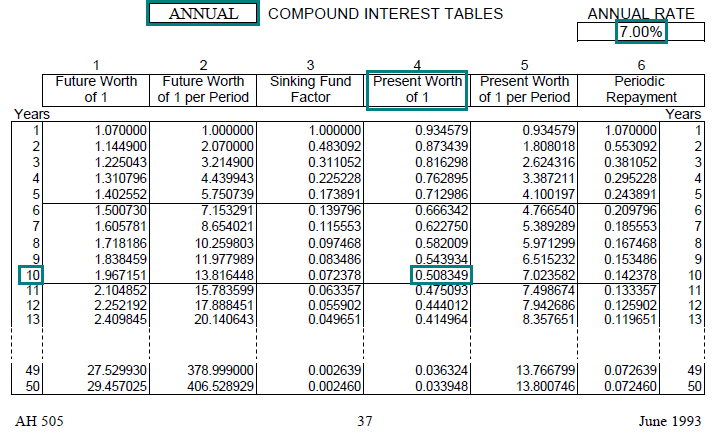Link to AH 505, page 37

Example 2:
Someone promises to pay you \$25,000 in 5 years. Given an annual interest rate of 6% with annual compounding, how much should you pay for this promise today?

Solution:

• PV = FV × PW\$1 (6%, 5 yrs, annual)
• PV = \$25,000 × 0.747258
• PV = \$18,681
• Find the annual PW\$1 factor (annual compounding) for 6% at a term of 5 years. In AH 505, page 33, go down 5 years and across to column 4 to find the correct factor of 0.747258.
• The present value of \$18,681 is equal to the future value of \$25,000 multiplied by the factor.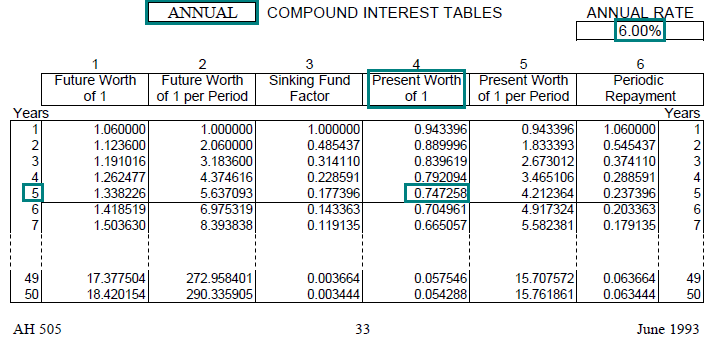Link to AH 505, page 33

Example 3:
If you want to have \$10,000 after 3 years, and you can invest at an annual rate of 5% compounded annually, how much should you invest today?

Solution:

• PV = FV × PW\$1 (5%, 3 yrs, annual)
• PV = \$10,000 × 0.863838
• PV = \$8,638
• Find the annual PW\$1 factor (annual compounding) for 5% at a term of 3 years. In AH 505, page 29, go down 3 years and across to column 4 to find the correct factor of 0.863838.
• The present value of \$8,638 is equal to the future value of \$10,000 multiplied by the factor.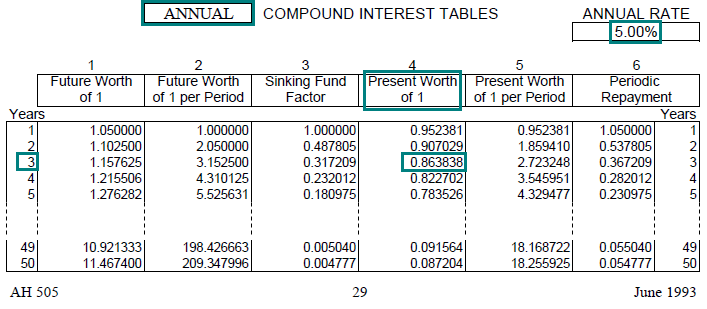Link to AH 505, page 29

Example 4:
Ten years from now, you will need to make a lump-sum payment of \$500,000. Assuming annual compounding, how much should you invest today in order to cover the future payment? The annual interest rate is 10%.

Solution:

• PV = FV × PW\$1 (10%, 10 yrs, annual)
• PV = \$500,000 × .385543
• PV = \$192,772
• Find the annual PW\$1 factor (annual compounding) for 10% at a term of 10 years. In AH 505, page 49, go down 10 years and across to column 4 to find the correct factor of 0.385543.
• The present value of \$192,772 is equal to the future value of \$500,000 multiplied by the factor.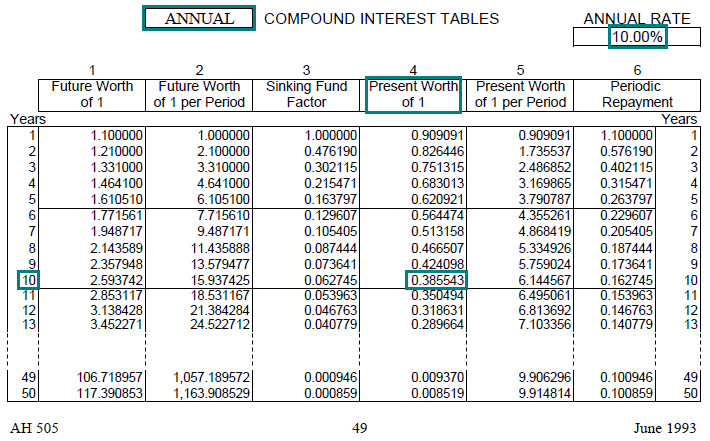Link to AH 505, page 49

Example 5:
Acme Enterprises promises to pay the holders of its most recent bond issue \$1,000 per bond at the end of 25 years (there are no annual or semi–annual interest payments; this is called a "zero coupon" bond). If the annual interest rate is 8.50%, assuming annual compounding, how much should each bond sell for when issued?

Solution:

• PV = FV × PW\$1 (8.50%, 25 yrs, annual)
• PV = \$1,000 × 0.130094
• PV = \$130.09
• Find the annual PW\$1 factor (annual compounding) for 8.50% at a term of 25 years. In AH 505, page 43, go down 25 years and across to column 4 to find the correct factor of 0.130094.
• The present value of \$130.09 is equal to the future value of \$1,000 multiplied by the factor. The bond should sell for \$130.09.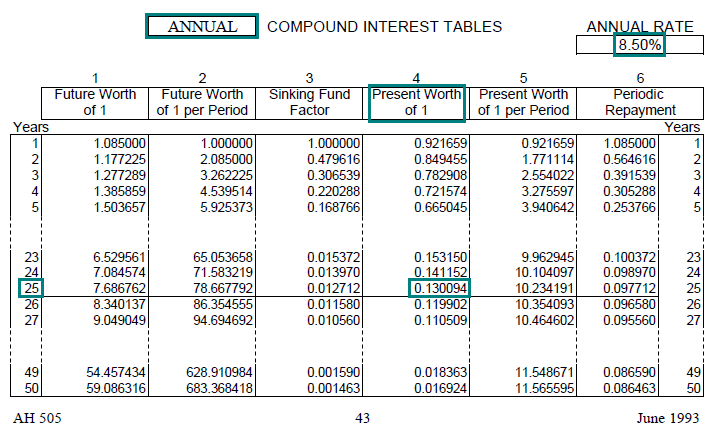Link to AH 505, page 43

## Multiple Payments and PW\$1

We have calculated the present value of single amounts or payments, using the PW\$1 factors.

Many problems involve more than one payment, making it necessary to calculate the present value of multiple payments–that is, the present value of a stream of payments. Determining the present value of multiple payments is a straightforward extension of the single-payment situation.

When we calculated the present value of a single future payment, we multiplied the future payment by the appropriate PW\$1 factor. This discounted the future payment to its present value.

If there is more than one future payment, multiple each payment by the appropriate PW\$1 factor and add the present values. The sum of the present values is the total present value of the stream of future payments.

## Practical Applications of PW\$1 with Multiple Payments

Example 1:
Consider the following 3 payments:

1. \$10,000 at the end of the first year
2. \$15,000 at the end of the second year
3. \$20,000 at the end of the third year

At an annual interest rate of 5%, what is the total present value of the 3 payments?

Solution:
Calculate the present value of each payment using the PW\$1 factors and add those present values. The sum is the present value of all 3 payments.

Thus: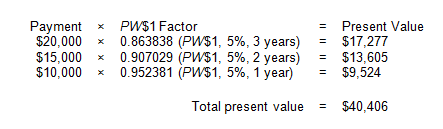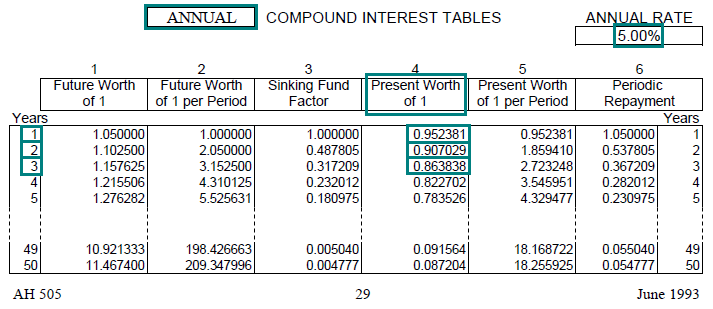Link to AH 505, page 29

Viewed on a timeline: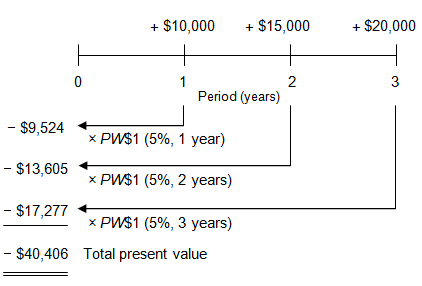A person would be willing to pay \$40,406 now (shown as negative on the timeline) in order to receive the three future payments of \$10,000, and \$15,000, and \$20,000 (shown as positive).